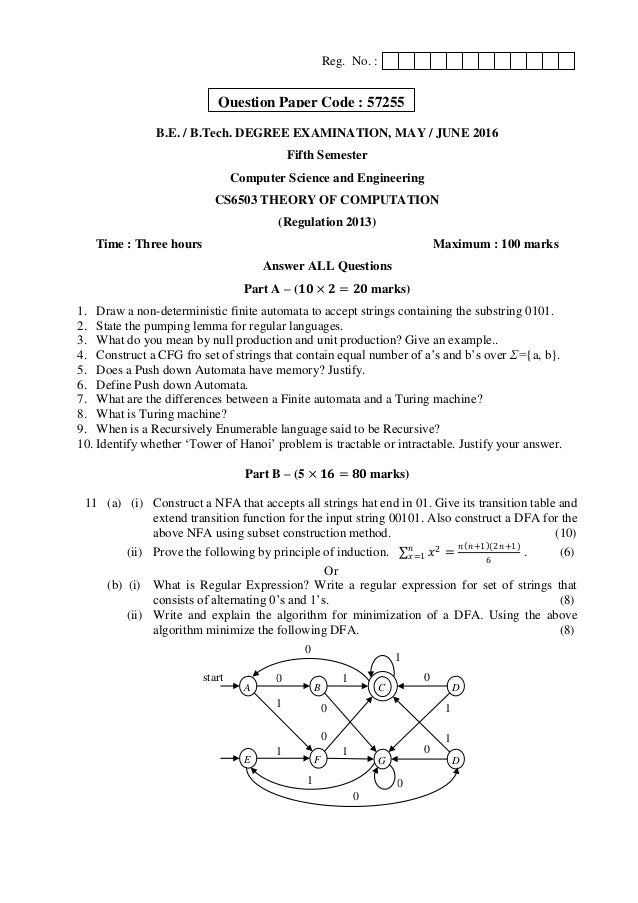Successfully reported this slideshow.
We use your LinkedIn profile and activity data to personalize ads and to show you more relevant ads. You can change your ad preferences anytime.Upcoming SlideShare
×

# Cs6503 theory of computation may june 2016 be cse anna university question paper

5,903 views

Published on

Cs6503 theory of computation may june 2016 be cse anna university question paper

Published in: Engineering
• Full Name
Comment goes here.

Are you sure you want to Yes No
Your message goes here• Be the first to comment

### Cs6503 theory of computation may june 2016 be cse anna university question paper

1. 1. Reg. No. : B.E. / B.Tech. DEGREE EXAMINATION, MAY / JUNE 2016 Fifth Semester Computer Science and Engineering CS6503 THEORY OF COMPUTATION (Regulation 2013) Time : Three hours Maximum : 100 marks Answer ALL Questions Part A – (𝟏𝟎 × 𝟐 = 𝟐𝟎 marks) 1. Draw a non-deterministic finite automata to accept strings containing the substring 0101. 2. State the pumping lemma for regular languages. 3. What do you mean by null production and unit production? Give an example.. 4. Construct a CFG fro set of strings that contain equal number of a‟s and b‟s over Σ={a, b}. 5. Does a Push down Automata have memory? Justify. 6. Define Push down Automata. 7. What are the differences between a Finite automata and a Turing machine? 8. What is Turing machine? 9. When is a Recursively Enumerable language said to be Recursive? 10. Identify whether „Tower of Hanoi‟ problem is tractable or intractable. Justify your answer. Part B – (5 × 𝟏𝟔 = 𝟖𝟎 marks) 11 (a) (i) Construct a NFA that accepts all strings hat end in 01. Give its transition table and extend transition function for the input string 00101. Also construct a DFA for the above NFA using subset construction method. (10) (ii) Prove the following by principle of induction. 𝑥2𝑛 𝑥=1 = 𝑛 𝑛+1 (2𝑛+1) 6 . (6) Or (b) (i) What is Regular Expression? Write a regular expression for set of strings that consists of alternating 0‟s and 1‟s. (8) (ii) Write and explain the algorithm for minimization of a DFA. Using the above algorithm minimize the following DFA. (8) A 0 1start B C D 0 1 0 E 1 1 F G D 0 0 01 1 1 1 0 0 Question Paper Code : 57255
2. 2. 12 (a) (i) Construct a reduced grammar equivalent to the grammar G = (N, T, P, S) where, N = {S, A, C, D, E} (6) T = {a, b} P = { S → aAa, A → Sb, A → bCC, A → DaA, C → abb, C → DD, E → aC, D → aDA}. (ii) When is a grammar said to be ambiguous? Explain with the help of example. (5) (iii) Show the derivation steps and construct derivation tree for the string „ababbb‟. (5) by using left most derivation with the grammar. S → AB | ε A→ aB B→ Sb Or (b) (i) What is the purpose of normalization? Construct the CNF and GNF for the following grammar and explain the steps. (10) S → aAa | bBb | ε A→ C | a B→ C | b C→ CDE | ε D→ A | B | ab (ii) Construct a CFG for the regular expression (011+1) (01). (6) 13 (a) (i) Construct a Push down Automata to accept the following language L on Σ={a, b} by empty stack. 𝐿 = {𝑤𝑤R | 𝑤 ∈ 𝛴+ }. (10) (ii) What is an instantaneous description that the PDA? How will you represent it? Also give three important principles of ID and their transactions. (6) Or (b) (i) Explain acceptance by final state and acceptance by empty stack of a Push down Automata. (8) (ii) State the pumping lemma for CFL. Use pumping lemma to show that the language 𝐿 = {𝑎i 𝑏j 𝑐k | 𝑖 < 𝑗 < 𝑘} is not a CFL. (8) 14 (a) (i) Construct a Turing Machine to accept palindromes in an alphabet set Σ={a, b}. Trace the string “abab” and “baab”. (8) (ii) Explain the variations of Turing Machine. (8) Or (b) (i) Explain Halting problem. Is it solvable or unsolvable problem? Discuss. (8) (ii) Describe the Chomsky hierarchy of languages with example. What are the devices that accept these languages? (8) 15 (a) What is Universal Turing Machine? Bring out its significance. Also construct a Turing Machine to add two numbers and encode it. (16) Or (b) What is post correspondence problem (PCP)? Explain with the help of an example. (16) All the Best – No substitute for hard work. Mr. G. Appasami SET, NET, GATE, M.Sc., M.C.A., M.Phil., M.Tech., (P.hd.), MISTE, MIETE, AMIE, MCSI, MIAE, MIACSIT, MASCAP, MIARCS, MISIAM, MISCE, MIMS, MISCA, MISAET, PGDTS, PGDBA, PGDHN, PGDST, (AP / CSE / DRPEC).